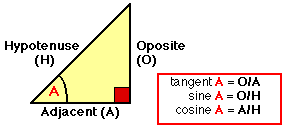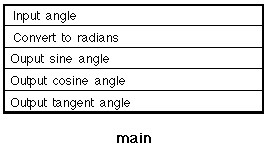# COMP101 EXAMPLE PROBLEM - SINE, COSINE AND TANGENT

## 1. REQUIREMENTS

Produce a Java class that will return the sine, cosine and tangent of a given angle between 0.0 and 90.0 degrees (Figure 1).

` `Figure 1: Sine, cosine and tangent trigonometric identities

## 2. ANALYSIS AND DESIGN

The design comprises a single class, TrigIdentitiesApp; all other methods used are contained in existing classes that come with the Java API.

### 2.1 TrigIdentitiesApp Class

A Nassi-Shneiderman presented in Figure 2.Fig 2: Nassi-Shneidermancharts for TrigIdentitiesApp class method

` `
 Field Summary private static Scanner keyboardInput            A class instance to facilitate input from the keyboard. private static final double DEC_2_RAD            A class constant facilitate conversion from degrees to radians.

 Method Summary public static void main(String[] args)            Allows user to input an angle (in degrees) which is then converted to radians and the appropriate values tangent, sine and cosine ratios produced.

### 3. IMPLEMENTATION

 ```// TRIGONOMETRIC IDENTITIES // Frans Coenen // Tuesday 12 March 1999 // Modified: Saturday 17 September 2005 (Java 2.5) // The University of Liverpool, UK import java.util.*; class TrigIdentitiesApp { // ------------------- FIELDS ------------------------ // Create BufferedReader class instance static Scanner keyboardInput = new Scanner(System.in); // Constants private static final double DEG_2_RAD = Math.PI/180.0; // ------------------ METHODS ------------------------ public static void main(String[] args) { double angle; // Input an angle System.out.print("Input an anagle between 0 and 90 " + "degrees inclusive: "); angle = keyboardInput.nextDouble(); // Convert to radians angle = angle*DEG_2_RAD; // Sine Angle System.out.println("Sine is: " + Math.sin(angle)); // Cosine Angle System.out.println("Cosine is: " + Math.cos(angle)); // Tangent Angle System.out.println("Tangent is: " + Math.tan(angle)); } } ```

Table 1: Trigonometric identities program

Note that the implementation given in Table 1 uses the constant, DEG_2_RAD, to convert input in degrees to radians.

### 4. TESTING

TEST CASEEXPECTED RESULT
e java.lang.NumberFormatException
10.e java.lang.NumberFormatException

Arithmetic testing: We have one input therefore we should test negative, zero and positive inputs. A suitable set of test cases is given in the table to the right. Note that we have include a number of positive values (30, 45, 60 and 90) because these have well known results.

` `
TEST CASE angle Sine EXPECTED RESULT -90 -1.00000 6.12303 -1.63318 0 0.00000 1.00000 0.00000 30 0.50000 0.86602 0.57735 45 0.70711 0.70711 1.00000 60 0.86602 0.50000 1.73205 90 1.00000 6.12303 1.63318

An alternative implementation is given in Table 2 where the toRadians class methhod from the Math is used.

 ```// TRIGONOMETRIC IDENTITIES VERSION 2 // Frans Coenen // Tuesday 12 March 1999 // Modified: Saturday 17 September 2005 (Java 2.5) // The University of Liverpool, UK import java.util.*; class TrigIdentitiesVer2App { // ------------------- FIELDS ------------------------ // Create BufferedReader class instance static Scanner keyboardInput = new Scanner(System.in); // Constants private static final double DEG_2_RAD = Math.PI/180.0; // ------------------ METHODS ------------------------ public static void main(String[] args) { double angle; // Input an angle System.out.print("Input an anagle between 0 and 90 " + "degrees inclusive: "); angle = keyboardInput.nextDouble(); // Convert to radians angle = Math.toRadians(angle); // Sine Angle System.out.println("Sine is: " + Math.sin(angle)); // Cosine Angle System.out.println("Cosine is: " + Math.cos(angle)); // Tangent Angle System.out.println("Tangent is: " + Math.tan(angle)); } } ```

Table 2: Alternative trigonometric identities program

Created and maintained by Frans Coenen. Last updated 17 September 2005